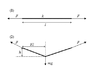# Streching rope

Paul L
The problem:
See attachment for figure.
(1) A rope with a spring constant k is stretched by a force F.The length of the rope is l (including the deformation).
(2) A force mg is then applied at l/2 on the rope. This results in a height difference between the highest (and unchanged point) and the lowest point, h. The length between the endpoints where the force F is applied is still l.

Equations:
Hooke's law for springs: F=kx
Maybe something else? Cant see where and what though...

The question:
Set up an equation for the force F.

My attempts:
I've tried to solve this problem with geometric similarity between the force and the deformation, but obviously not correctly...

Applying in real life:
This is thought to be a model of a slack line set up. If you don't know what that is, do a search on flickr.com and you'll get the idea. Is it reasonable to expect that such thing will follow Hooke's law for springs? Is there any other theory that is better to use? Links?

#### Attachments

•rope.GIF
2 KB · Views: 435

Homework Helper
F in the figure 1 is not equal to F in the figure 2.
F in the figure 2 can be found in terms of mg by using vector method.
New half length of the string can be found in terms of l/2 and h.
Using these values you can find F in figure 1.

Homework Helper
L/h = S/F This is not correct. It should be L/(l/2) = S/F and h/L = mg/2S. Solve for F.

Paul L
I'm not sure if I'm following you, but I tried what you suggested (attachment once again) and ended up with this:

F=(mgl)/(4h)

I have a bit problems believing that's correct as it would mean that an inelastic rope (k -> infinity) will stretch and bend down equally much as a elastic rope when the same force is applied.

#### Attachments

Homework Helper
For a perfecly elestic body k -> infinity and for a perfectly inelastic body k->0. If you replace the rope by a steel rod and apply the force mg at the mid point, the depression of the mid point is very small.

Paul L
For a perfecly elestic body k -> infinity and for a perfectly inelastic body k->0. If you replace the rope by a steel rod and apply the force mg at the mid point, the depression of the mid point is very small.

For a perfectly inelastic body k -> infinity. Hooke's law gives x = F/k and F/k -> 0 when k -> infinity. For a perfectly inelastic body, x = 0 for any given force F.

If the rope is replaced with a steel rod this would be a completely different problem as a rod can absorb torque and a rope cannot. However if you replace the rope with a steel wire, the depression would indeed be very small. That's why the equation F=(mgl)/(4h) can't be correct as it doesn't take this into account.

I still haven't figured out this problem, so any help would be highly appreciated.

Homework Helper
Young's modulus of elesticity is given by Y = Stress / strain = (F/A)/(x/L) where F is the stretching force, A is the area of cross section, x is th elongation and L is the original length. So F = [(y*A)/L]*x or F = k*x So for a given deforming force F, when K...> infinity x...> zero. When you apply froce S, x increases.( since k remains constant) And hence the depression of the mid point h increases. Steel is more elestric than rubber, because for an applied force elongation in steel wire is very small compared to that in rubber.

Paul L
Steel is more elestric than rubber, because for an applied force elongation in steel wire is very small compared to that in rubber.

And therefore should the material constant k be included in the equation when the depression (and hence the elongation) is given. The equation F=(mgl)/(4h) must be wrong since it doesn't include the material constant.

Homework Helper
Welcome to PF!

Hi Paul! Welcome to PF!What a mess everyone is making!This is a perfectly simple force diagram question …

it's a rope, so the only force in it is the tension …

you can work out the tension from the length, or vice versa

and the tension is the same at each end …

and you know the angle …

so … ?